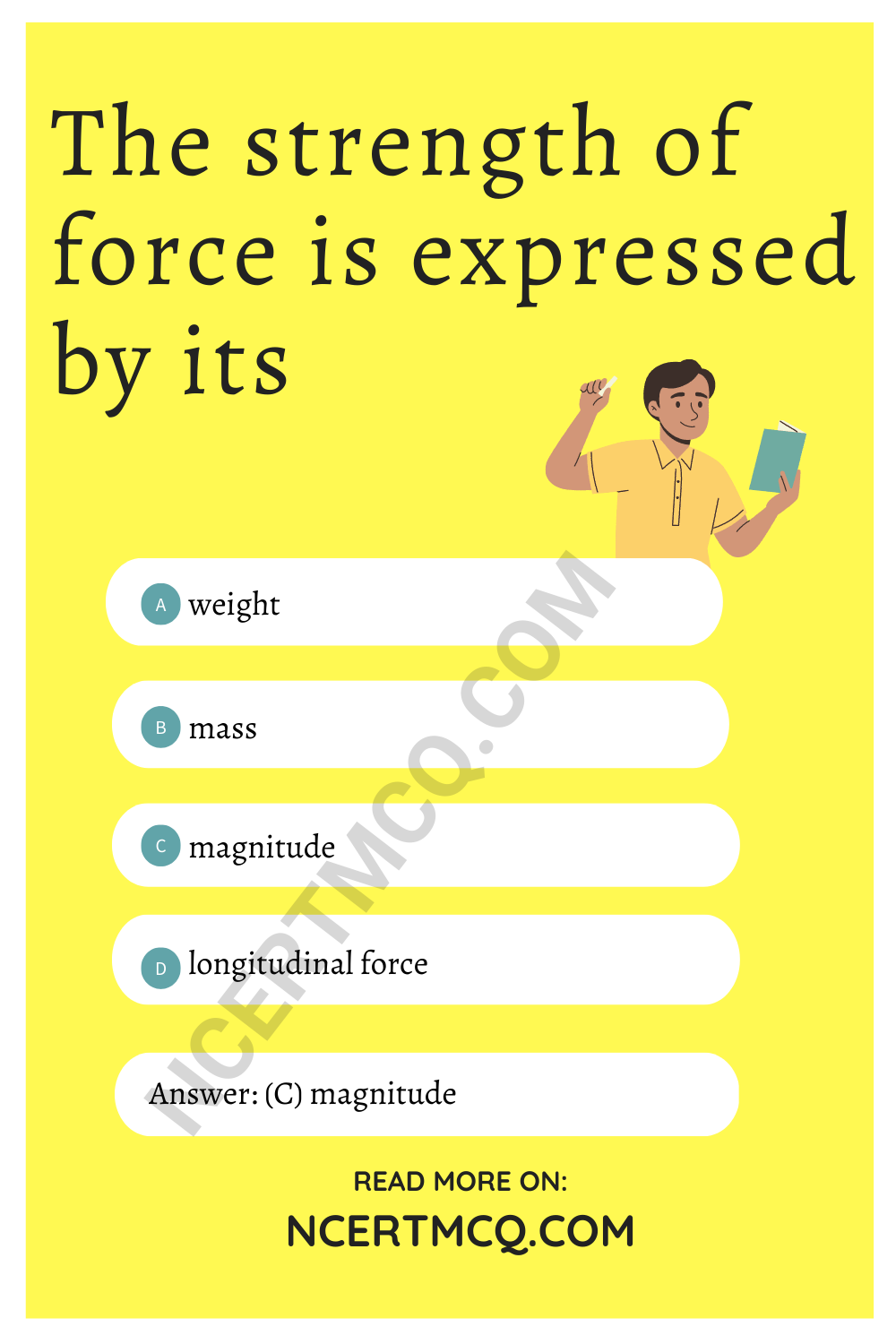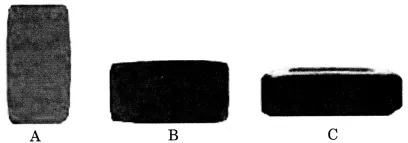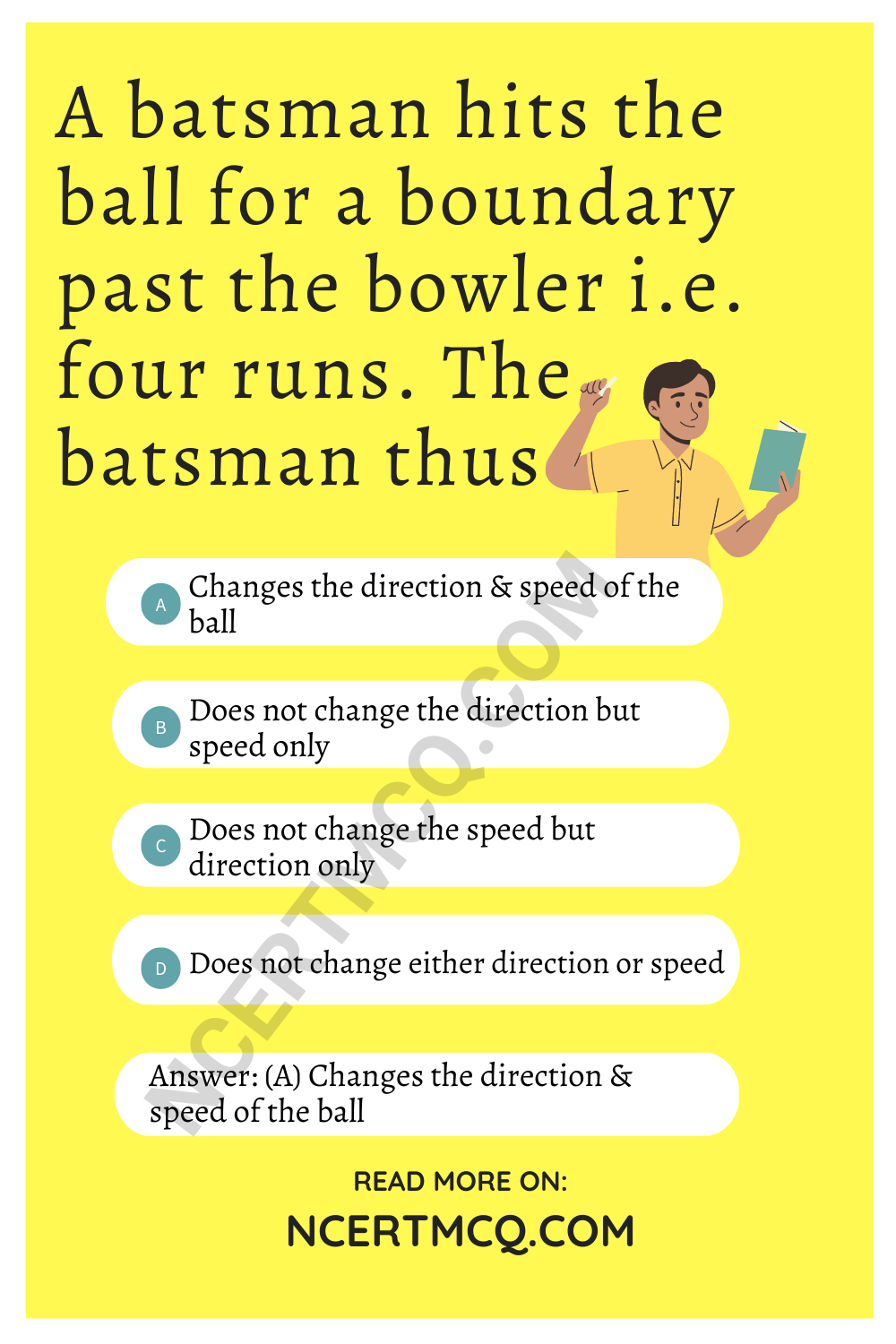Check the below Online Education NCERT MCQ Questions for Class 8 Science Chapter 11 Force and Pressure with Answers Pdf free download. MCQ Questions for Class 8 Science with Answers were prepared based on the latest exam pattern. We have provided Force and Pressure Class 8 Science MCQs Questions with Answers to help students understand the concept very well. https://ncertmcq.com/mcq-questions-for-class-8-science-with-answers/

You can refer to NCERT Solutions for Class 8 Science Chapter 11 Force and Pressure to revise the concepts in the syllabus effectively and improve your chances of securing high marks in your board exams.

## Force and Pressure Class 8 MCQs Questions with Answers

Choose the correct option.

Force And Pressure Class 8 MCQ Question 1.
The pressure which is exerted by air around us is known as
(a) force
(b) atmospheric pressure
(c) muscular force
(d) friction

Class 8 Science Chapter 11 MCQ Question 2.
Force acting on per unit area is called
(a) non-contact forces
(b) contact forces
(c) force
(d) pressure

Class 8 Force And Pressure MCQ Question 3.
A ___________ exerted by an object on another is a force.
(a) Push or pull
(b) Contact or non-contact force
(c) Pressure
(d) Magnitude

MCQ On Force And Pressure Class 8 Question 4.
The force exerted by the earth to pull the object towards itself is called
(a) electrostatic force
(b) gravitational force
(c) muscular force
(d) contact force

Class 8 Science Ch 11 MCQ Question 5.
Muscular force is also called ___________ force.
(a) non-contact
(b) contact
(c) gravitational
(d) magnetic

MCQ Of Force And Pressure Class 8 Question 6.
The force exerted by a charged body on another char
(a) gravitational force
(b) electrostatic force
(c) non-contact force
(d) contact force

Ch 11 Science Class 8 MCQ Question 7.
Force changes the
(a) motion of body
(b) speed of body
(c) shape of body
(d) all of these

MCQ Questions For Class 8 Science Chapter 11 Question 8.
The force exerted by our muscle is called
(a) electrostatic force
(b) muscular force
(c) gravitational force
(d) non-contact force

Force And Pressure MCQ Class 8 Question 9.
1 kilogram weight is equal to
(a) 98 N
(b) 9.8 N
(c) 0.98 N
(d) 0.098 N

Class 8 Chapter 11 Science MCQ Question 10.
A spring balance is used for measuring
(a) mass
(b) weight
(c) pressure
(d) speed

Class 8 Science Force And Pressure MCQ Question 11.
Two boys A and B are applying force on a block. If the block moves towards the boy A, which one of the following statements is correct?
(a) Magnitude of force applied by A is greater than that of B.
(b) Magnitude of force applied by A is smaller than that of B.
(c) Net force on the block is towards B.
(d) Magnitude of force applied by A is equal to that of B.

Answer: (b) Magnitude of force applied by A is smaller than that of B.

Force And Pressure Class 8 MCQ With Answers Question 12.
When two forces act in opposite directions, then net force acting is the
(a) sum of two forces
(b) difference between two forces
(c) both of these
(d) none of these

Answer: (b) difference between two forces

Ncert Class 8 Science Chapter 11 MCQ Question 13.
The strength of force is expressed by its
(a) weight
(b) mass
(c) magnitude
(d) longitudinal forceMCQ Force And Pressure Class 8 Question 14.
Leaves fall down on the ground due to
(a) electrostatic force
(b) magnetic force
(c) gravitational force
(d) muscular force

Class 8 Ch 11 Science MCQ Question 15.
State of motion is described by
(a) Position of rest
(b) Position of motion
(c) Both by the state of rest or motion
(d) None of these

Answer: (c) Both by the state of rest or motion

Question 16.
When the hammer strikes the gong of an electric bell, which of the following force is responsible for the movement of hammer?
(a) Gravitational force alone
(b) Magnetic force alone
(c) Electrostatic force alone
(d) Frictional force alone

Question 17.
During dry weather, while combing hair, sometimes we experience hair flying apart. The force respon¬sible for this is
(a) force of gravity
(b) force of friction
(c) electrostatic force
(d) magnetic force

Question 18.
A container is filled with water as shown in the given figure. Which of the following statements is correct about pressure of water?(a) Pressure at A > Pressure at B > Pressure at C
(b) Pressure at A = Pressure at B = Pressure at C
(c) Pressure at A < Pressure at B > Pressure at C
(d) Pressure at A < Pressure at B < Pressure at C

Answer: (d) Pressure at A < Pressure at B < Pressure at C

Question 19.
Two objects repel each other. This repulsion could be due to
(a) frictional force only
(b) electrostatic force only
(c) magnetic force only
(d) either a magnetic or an electrostatic force

Answer: (d) either a magnetic or an electrostatic force

Question 20.
Which one of the following forces is a contact force?
(a) Force of gravity
(b) Magnetic force
(c) Force of friction
(d) Electrostatic force

Question 21.
A brick is kept in three different ways on a table as shown in given figure. The pressure exerted by the brick on the table will be(a) maximum in position A
(b) maximum in position C
(c) maximum in position B
(d) equal in all cases

Answer: (a) maximum in position A

Question 22.
A push or pull on an object is called
(a) Pressure
(b) Push-pull
(c) Force
(d) All of the above

Question 23.
If two forces act in the opposite directions on an object, the net force acting on it is the
(a) Sum of the two forces
(b) difference between the two forces
(c) Multiplication of the two forces
(d) Division of the two forces

Answer: (b) difference between the two forces

Question 24.
Which of the following is proper example(s) to explain that force on an object may change its shape
(a) A ball of dough rolled into chapati
(b) Pressing a rubber ball kept on table
(c) Making model using clay
(d) All of the above

Answer: (d) All of the above

Question 25.
A ball rolling on the ground slows down and finally stops. This is because of
(a) Force
(b) Less force applied
(c) Friction
(d) None of the above

Question 26.
Force of friction always acts on moving objects and its direction shall be ____
(a) On any direction
(b) Along the direction of motion
(c) Perpendicular to the direction of motion
(d) Opposite to the direction of motion

Answer: (c) Perpendicular to the direction of motion

Question 27.
If In a tug-o-war, when two teams are pulling a rope, and the rope does not move towards any team, it implies that
(a) Equal force is being applied in the same direction
(b) Equal Force is being applied in opposite direction
(c) No force is applied in any direction
(d) Cannot be explained

Answer: (b) Equal Force is being applied in opposite direction

Question 28.
An example of a non- contact force is
(a) Force exerted by us to lift a bucket
(b) Push a stationary car
(c) Force exerted by magnet
(d)  Hit a cricket ball for a 6 run

Answer: (c) Force exerted by magnet

Question 29.
Pressure =
(a) Area / force on which it acts
(b) force / area on which it acts
(c) Volume / force on which it acts
(d) Force / volume on which it acts

Answer: (b) force / area on which it acts

Question 30.
Gravity is
(a) Repulsive
(b) Attraction + Repulsive force
(c) Attractive force
(d) Not a force

Question 31.
A batsman hits the ball for a boundary past the bowler i.e. four runs. The batsman thus
(a) Changes the direction & speed of the ball
(b) Does not change the direction but speed only
(c) Does not change the speed but direction only
(d) Does not change either direction or speed

Answer: (a) Changes the direction & speed of the ballMatch the items given in column I suitably with those given in column II.

 Column I Column II 1. Pressure (a) Fluid pressure 2. Barometer (b) Unit of pressure 3. Newton (c) Non-contact force 4. Manometer (d) Force/Area 5. Pascal (e) Unit of force 6. Magnetic force (f) Net force acting on a body 7. 1 kilogram (g) Atmospheric pressure 8. Resultant force (h) 9.8 N 9. Frictional force (i) Down the column 10. Pressure increases (j) Contact force

 Column I Column II 1. Pressure (d) Force/Area 2. Barometer (g) Atmospheric pressure 3. Newton (e) Unit of force 4. Manometer (a) Fluid pressure 5. Pascal (b) Unit of pressure 6. Magnetic force (c) Non-contact force 7. 1 kilogram (h) 9.8 N 8. Resultant force (f) Net force acting on a body 9. Frictional force (j) Contact force 10. Pressure increases (i) Down the column

Fill in the blanks with suitable word/s.

1. We ___________ the bucket to take out water from well.

2. The strength of a force is usually expressed by its ___________.

3. ___________ force is the force resulting due to the action of muscles.

4. The envelop of air all around us is called ___________.

5. To open a door we or ___________ the door.

6. Force of friction is an example of ___________ force.

7. Force ___________ is called pressure.

8. Force has ___________ as well as direction.

9. Fruits fall ___________ due to the force of gravity.

10. The body at rest is called ___________ body.

11. ___________ and ___________ forces are the two kinds of forces.

12. Force exerted by magnet is called ___________ force.

13. We apply force on ___________ while walking.

14. Force is ___________ to pressure.

15. The ___________ is measured by an instrument called barometer.

State whether the given statements are true or false.

1. Force applied on an object in opposite directions add to one another.

2. Pressure does not depend on area of contact.

3. A force can change the state of motion of an object.

4. Force cannot change the speed of an object.

5. Atmospheric pressure is less at higher altitudes.

6. Force of gravity is a contact force.

7. Muscular force is also known as contact force.

8. Pascal is the unit of force.

9. To move an object faster it has to be pushed or pulled repeatedly.

10. Magnitude is the strength of force.

11. Force can change the shape of an object.

12. At least two objects must interact for a force to come into play.

13. Magnetic force is a non-contact force.

14. An apple from a tree falls on the ground due to the force of gravity.